Processing ......FreeComputerBooks.com Links to Free Computer, Mathematics, Technical Books all over the World

Geometry and Topology
Related Book Categories:
•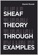Sheaf Theory through Examples (Daniel Rosiak)

Taking an applied category theory perspective, this book provides an approachable introduction to elementary sheaf theory and examines applications. It seeks to bridge the powerful results of sheaf theory as used by mathematicians and real-world applications,

•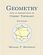Geometry with an Introduction to Cosmic Topology

The text uses Mobius transformations in the extended complex plane to define and investigate these three candidate geometries, thereby providing a natural setting in which to express results common to them all, as well as results that encapsulate key differences.

•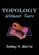Topology without Tears (Sidney A. Morris)

General topology is the branch of topology dealing with the basic set-theoretic definitions and constructions used in topology. The aim of this is to provide a thorough grouding of general topology. It offers an ideal introduction to the fundamentals of topology.

•Topological Groups: Yesterday, Today, Tomorrow (Sidney A. Morris)

In 1900, David Hilbert asked whether each locally euclidean topological group admits a Lie group structure. This was the fifth of his famous 23 questions which foreshadowed much of the mathematical creativity of the twentieth century.

•Topology: A Categorical Approach (Tai-Danae Bradley, et al)

A graduate-level textbook that presents basic topology from the perspective of category theory. Many graduate students are familiar with the ideas of point-set topology and they are ready to learn something new about them.

•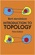Introduction to Topology (Bert Mendelson, et al.)

This concise book offers an ideal introduction to the fundamentals of topology. The book's principal aim is to provide a simple, thorough survey of elementary topics in the study of collections of objects, or sets, that possess a mathematical structure.

•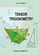Tensor Trigonometry (A.S. Ninul)

The tensor trigonometry is development of the flat scalar trigonometry from Leonard Euler classic forms into general multi-dimensional tensor forms with vector and scalar orthoprojections and with step by step increasing complexity and opportunities.

•Elementary College Geometry (Henry Africk)

This text is intended for a brief introductory course in plane geometry, covers the topics from elementary geometry that are most likely to be required for more advanced mathematics courses. The only prerequisite is a semester of algebra.

•Differential Geometry: A Geometric Introduction

This is the only book that introduces differential geometry through a combination of an intuitive geometric foundation, a rigorous connection with the standard formalisms, computer exercises with Maple, and a problems-based approach.

•Computational Linear Algebra and N-dimensional Geometry

This undergraduate textbook on Linear Algebra and n-Dimensional Geometry, in a self-teaching style, is invaluable for sophomore level undergraduates in mathematics, engineering, business, and the sciences.

•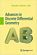Advances in Discrete Differential Geometry (Alexander I. Bobenko)

It surveys the fascinating connections between discrete models in differential geometry and complex analysis, integrable systems and applications in computer graphics and is illustrated to convince readers it's both beautiful and useful.

•Manifolds - Current Research Areas (Paul Bracken)

This book cover a number of subjects which will be of interest to workers in these areas. It is hoped that the papers here will be able to provide a useful resource for researchers with regard to current fields of research in this important area.

•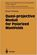Quasi-projective Moduli for Polarized Manifolds (Eckart Viehweg)

This book discusses two subjects of quite different nature: Construction methods for quotients of quasi-projective schemes by group actions or by equivalence relations and properties of direct images of certain sheaves under smooth morphisms.

•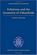Foliations and the Geometry of 3-Manifolds (Danny Calegari)

This book is to expose the "pseudo-Anosov" theory of foliations of 3-manifolds, which generalizes Thurston's theory of surface automorphisms, and reveals an intimate connection between dynamics, geometry and topology in 3 dimensions.

•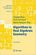Algorithms in Real Algebraic Geometry (Saugata Basu, et al)

The monograph gives a self-contained detailed exposition of the algorithmic real algebraic geometry. It will be useful both for beginners and for advanced readers, who work in real algebraic geometry or apply its methods in other fields.

•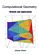Computational Geometry: Methods and Applications (Jianer Chen)

An introduction to some of the fundamental principles of computational geometry, concentrates on four major directions in computational geometry: the construction of convex hulls, proximity problems, searching problems and intersection problems.

•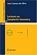Lectures on Symplectic Geometry (Ana Cannas da Silva)

The goal of these notes is to provide a fast introduction to symplectic geometry for graduate students with some knowledge of differential geometry, de Rham theory and classical Lie groups.

•Fractal Geometry: Mathematical Foundations and Applications

This book has become a seminal text on the mathematics of Fractals. It introduces the general mathematical theory and applications of fractals in a way that is accessible to students from a wide range of disciplines.

•Fractal Geometry (Mark McClure, et al.)

Fractal geometry is a new way of looking at the world. This book combines text and graphics to offer the most accessible amount that any reader is likely to find, helping in the overall move toward scientific literacy.

•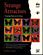Strange Attractors: Creating Patterns in Chaos (Julien C. Sprott)

This book describes a simple method for generating an endless succession of beautiful Fractal patterns by iterating simple maps and ordinary differential equations with coefficients chosen automatically by the computer.

•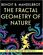The Fractal Geometry of Nature (Benoit Mandelbrot)

Explore the wondrously complex repeating shapes of the natural world in The Fractal Geometry of Nature. Written in a style that is accessible to a wide audience, computer scientist, professor, mathematician, etc.

•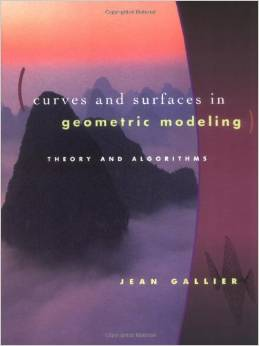Curves and Surfaces in Geometric Modeling: Theory and Algorithms

This book offers both a theoretically unifying understanding of polynomial curves and surfaces and an effective approach to implementation. It is also an exellent introduction to geometry concepts used in computer graphics, vision, robotics, geometric modeling.

•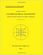Combinatorial Geometry with Application to Field Theory

Topics covered in this book include fundamental of combinatorics, algebraic combinatorics, topology with Smarandache geometry, combinatorial differential geometry, combinatorial Riemannian submanifolds, Lie multi-groups, etc.

•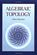Algebraic Topology (Allen Hatcher)

This introductory text is suitable for use in a course on the subject or for self-study, featuring broad coverage and a readable exposition, with many examples and exercises. The four main chapters present the basics: fundamental group and covering spaces, homology and cohomology, higher homotopy groups, and homotopy theory generally.

•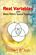Real Variables with Basic Metric Space Topology (Robert B. Ash)

Designed for a first course in real variables, this text presents the fundamentals for more advanced mathematical work, particularly in the areas of complex variables, measure theory, differential equations, functional analysis, and probability.

•Classical Algebraic Geometry: A Modern View (Igor V. Dolgachev)

This detailed exposition makes the rich legacy of classical algebraic geometry accessible to modern algebraic geometers and to others who are interested in applying classical results. Topics include plane algebraic curves of low degree, etc.

•Higher Topos Theory (Jacob Lurie)

This book presents the foundations of Higher Topos Theory, using the language of weak Kan complexes, and shows how existing theorems in algebraic topology can be reformulated and generalized in the theory's new language.

•Categorical Homotopy Theory (Emily Riehl)

This book develops abstract homotopy theory from the categorical perspective with a particular focus on examples. It helps consolidate and simplify one's understanding of derived functors, homotopy limits and colimits, and model categories, among others.

•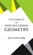The Elements of Non-Euclidean Geometry (Julian L. Coolidge)

The heroic age of non-euclidean geometry is passed. It is long since the days when Lobatchewsky timidly referred to his system as an 'imaginary geometry', and the new subject appeared as a dangerous lapse from the orthodox doctrine of Euclid.

•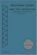Euclidean Plane and its Relatives: A Minimalist Introduction

The book is designed for a semester-long course in Foundations of Geometry and meant to be rigorous, conservative, elementary and minimalist. It promotes the art and the skills of developing logical proofs.

•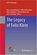The Legacy of Felix Klein (Hans-Georg Weigand, et al)

This open access book provides an overview of Felix Klein's ideas. It discusses the meaning, importance and the legacy of Klein's ideas today and in the future, within an international, global context.

•Convex Bodies and Algebraic Geometry: Theory of Toric Varieties

This book is an accessible introduction to current algebraic geometry of toric varieties gives new insight into continued fractions as well as their higher-dimensional analogues, the isoperimetric problem and other questions on convex bodies.

•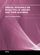Orbital Integrals on Reductive Lie Groups and Their Algebras

This book is to present a complete course on global analysis topics and establish some orbital applications of the integration on topological groups and their algebras to harmonic analysis and induced representations in representation theory.

•Probability on Trees and Networks (Russell Lyons, et al)

This book is concerned with certain aspects of discrete probability on infinite graphs that are currently in vigorous development. Of course, finite graphs are analyzed as well, but usually with the aim of understanding infinite graphs and networks.

•Art Gallery Theorems and Algorithms (Joseph O'Rourke)

This book is the most comprehensive collection of results on polygons currently available and thus earns its place as a standard text in discrete and computational geometry. It is very well written and a pleasure to read.

•Shape Interrogation for Computer Aided Design and Manufacturing

This book provides the mathematical fundamentals as well as algorithms for various shape interrogation methods including nonlinear polynomial solvers, intersection problems, differential geometry of intersection curves, distance functions etc.

•Algorithms for Modular Elliptic Curves, 2nd Edition (J. E. Cremona)

This book presents a thorough treatment of many algorithms concerning the arithmetic of elliptic curves, with remarks on computer implementation. An extensive set of tables is provided giving the results of the author's implementation of the algorithms.

•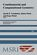Combinatorial and Computational Geometry (Jacob E. Goodman)

It includes surveys and research articles exploring geometric arrangements, polytopes, packing, covering, discrete convexity, geometric algorithms and their complexity, and the combinatorial complexity of geometric objects, particularly in low dimension.

•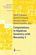Computations in Algebraic Geometry with Macaulay 2 (D.Eisenbud)

This book presents algorithmic tools for algebraic geometry and experimental applications of them using Macaulay 2. It also introduces a software system in which the tools have been implemented and with which the experiments can be carried out.

•Model Theory, Algebra, and Geometry (Deirdre Haskell, et al)

The book begins with an introduction to model theory. It then broadens into three components: pure model theory, geometry, and the model theory of fields. discussing the diverse areas of geometry (algebraic, diophantine, real analytic, p-adic, and rigid) to which the model theory is applied.

•Euclid and His Twentieth Century Rivals (Nathaniel Miller)

Twentieth-century developments in logic and mathematics have led many people to view Euclid's proofs as inherently informal, especially due to the use of diagrams in proofs. It introduces a diagrammatic computer proof system, based on this formal system.

•3D Math Primer for Graphics and Game Development (F. Dunn)

Working C++ classes for mathematical and geometric entities and several different matrix classes illustrate how to put the techniques into practice, and exercises at the end of each chapter help reinforce the 3D programming concepts.

•Vector Math for 3D Computer Graphics, 4th Revision (Bradley Kjell)

This is a tutorial on vector algebra and matrix algebra from the viewpoint of computer graphics. It covers most vector and matrix topics needed to read college-level computer graphics text books.

•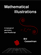Mathematical Illustrations: A Manual of Geometry and PostScript

It combines a completely self-contained step-by-step introduction to the graphics programming language PostScript with an analysis of the requirements of good mathematical illustrations, for anyone with basic knowledge of coordinate geometry.

•Topology of Numbers (Allen Hatcher)

A textbook on elementary number theory from a geometric point of view, as opposed to the usual strictly algebraic approach. A fair amount of the book is devoted to studying Conway's topographs associated to quadratic forms in two variables.

•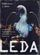LEDA: A Platform for Combinatorial and Geometric Computing

LEDA is a library of efficient data types and algorithms and a platform for combinatorial and geometric computing. This book, written by the main authors of LEDA, is the definitive account of how the system operates and how it can be used.

•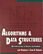Algorithms and Data Structures: Apps to Graphics and Geometry

An introductory coverage of algorithms and data structures with application to graphics and geometry aims to show a sample of the intellectual demands required by a computer science curriculum, exercises, many with solutions, are included throughout the book.

•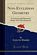Non-Euclidean Geometry: A Critical and Historical Study

This work has been selected by scholars as being culturally important, and is part of the knowledge base of civilization as we know it. This work was reproduced from the original artifact, and remains as true to the original work as possible.

•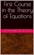First Course in the Theory of Equations (Leonard Dickson)

The theory of equations is not only a necessity in the subsequent mathematical courses and their applications, but furnishes an illuminating sequel to geometry, algebra and analytic geometry. A useful supplement to differential calculus.

Book Categories
 :All CategoriesTop Free BooksRecent BooksMiscellaneous BooksComputer EngineeringComputer LanguagesComputer ScienceData Science/DatabasesJava and Java EE (J2EE)Linux and UnixMathematicsMicrosoft and .NETMobile ComputingNetworking and CommunicationsSoftware EngineeringSpecial TopicsWeb Programming
Other Categories